# Berendsen thermostat

The Berendsen thermostat  is a method for controlling the temperature in a molecular dynamics simulation. The Berendsen thermostat uses a weak coupling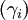$(\gamma_i)$ to an external heat bath of temperature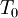$T_0$. This results in the modified equation of motion (Ref. 1 Eq. 8):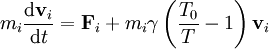$m_i \frac{{\mathrm d} {\mathbf {v}}_i}{{\mathrm d} t} = {\mathbf {F}}_i + m_i \gamma \left( \frac{T_0}{T} -1\right){\mathbf {v}}_i$.
This represents a proportional scaling of the velocities per time step from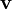${\mathbf {v}}$ to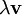$\lambda {\mathbf {v}}$, where (Ref. 1 Eq. 11)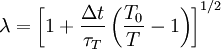$\lambda = \left[1 + \frac{\Delta t}{\tau_T} \left( \frac{T_0}{T} -1\right)\right]^{1/2}$
where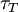$\tau_T$ is a time constant associated with the coupling.